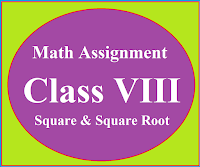### Math Assignment Class VIII | Square & Square RootMath Assignment  Class VIII | Square & Square Root Download or Print free  assignment with answer key  for   Class  8 Squares and  Square Roots.   Important and extra questions that cover all topics of square and square root and is useful and helpful for the students. Math Assignment  Class VIII | Square & Square Root LEVEL -1

CBSE mathematics  for 7th, 8th, 9th, 10th 11th and 12th classes. Maths formulas for the students, Step by step maths basic concepts for the students. Maths practical and project work for the students.

Reading and teaching mathematics is a challenge for the people. The main reason here is that most of the people read mathematics but they don't have interest in it. Without interest students cannot read mathematics for longer time so they decided to quit. If a student study mathematics step by step then surely the subject become interested for him. Through this blog we will try our best to reach the needs of the students and make mathematics interesting and valuable to them

MATHEMATICS RESOURCE CENTRE

Mathematics resource centre contains chapter-wise mathematics formulas worksheets, assignments, lesson plans, MCQ and case studies on mathematics and many other important links.

## By clicking on the link below students are able to find all that material which is helpful to learn and understand mathematics up to 12 standard. Through these links students can find the complete NCERT Solution of mathematics along with mathematics formulas and basic points  and chapter-wise mathematics assignments.

Students of 11th and 12th standard  click here to get Complete NCERT Sol. Chapter wise maths formula and Assignment.

## Lab Mannual or Lab Activities

Students click on the link below to find the

General Mathematics for all levels.

Mathematics Lab Activities(Lab Manual) for Class XI

Mathematics Lab Activities(Lab Manual) for Class XI

## E-Lesson Plan for Mathematics Teachers

For a teacher it is important to make a lesson plan. In mathematics lesson planning is an art. With the help of good planning a teacher can achieve his goal in the classroom. In the link given below teacher can find beautiful lesson planning in mathematics.

## Mathematics Assignments

Here systematic maths assignment along with the answers and solution hints for difficult questions  are given. Students can find variety of questions here.

1.2.1.